#### How to Write to a Cell in Excel Vba

In this Excel tutorial lesson, I will guide you through the basics of Excel VBA and how you can use Excel VBA to write to a cell in an Excel sheet.

## Recording a macro

Let’s start by recording macro. Go to the Ribbon to the Developer tab. Click the Record Marco button. Then click Edit. It will open the recorded macro as macro1 already created: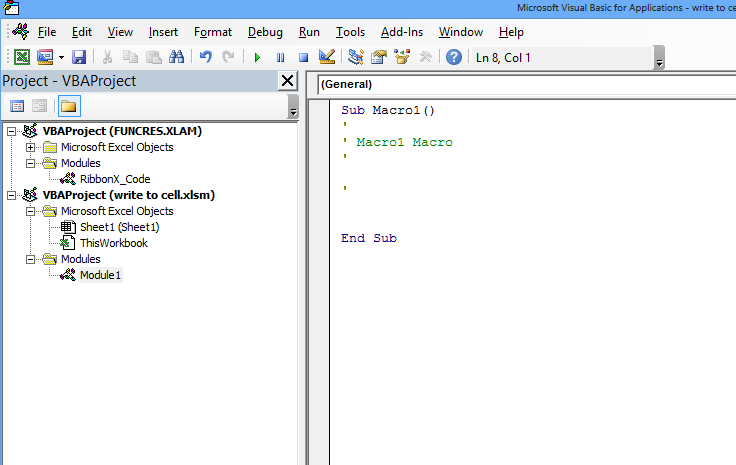Now under this module, you can learn how to write any information to a cell say cell A1 of sheet1. Here are following way you can update value in cell:

## Writing to a cell using worksheet

`ThisWorkbook.Sheets("Sheet1").Range("A1").Value = "hello"`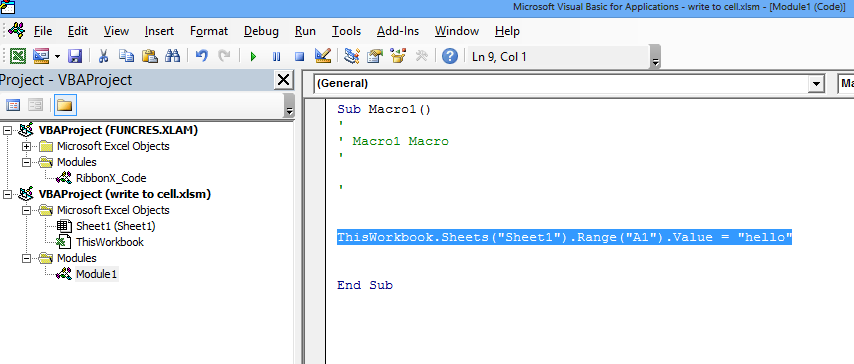Here “Sheet1” is the name of the sheet that you have used.

Run this macro: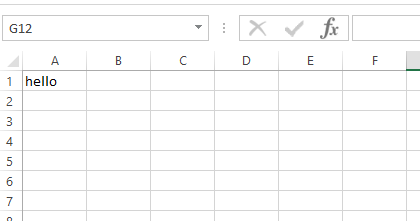## Writing to a cell using index number of sheet

`ThisWorkbook.Sheets(1).Range("A1").Value = "hello"`

Here you are referencing the sheet using the index, which is 1 for sheet1. You can also check the total count of sheets in a workbook using sheets.count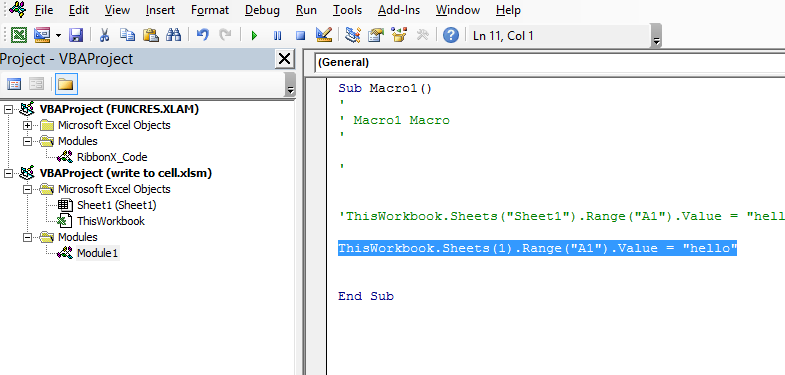## Writing to a cell using code name

`Sheet1.Range("A1").Value = "hello"`

You are directly using sheet1 which is the sheet name here.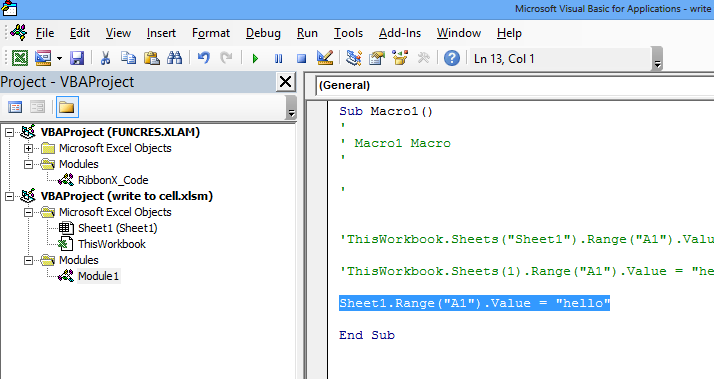## Writing to a cell using cells

` ThisWorkbook.Sheets(1).Cells(1, 1).Value = "hello"`

Instead of the range, you are using cells (row no, column no) where row no = 1 for the first row, column no = 1 for column A.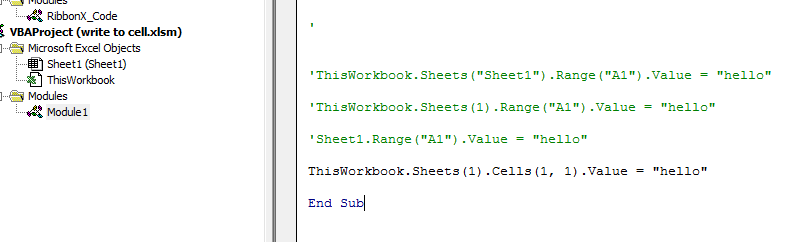## Writing a formula to a cell

`Range("A6").Formula = "=SUM(A3:A5)"`

This code writes the formula `=SUM(A3:A5)` to cell A6 in the active worksheet. The formula will calculate the sum of the values in cells A3 to A5.